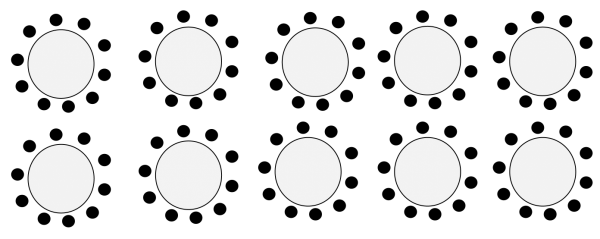## The Reflective Educator

### Education ∪ Math ∪ Technology

Close

I’m hoping to find (or potentially build, given how well my search is going) some open-ended problems appropriate for elementary school math classes. By open-ended problems, I mean problems which:

• do not have an obvious solution,
• require some time to figure out,
• have multiple solutions,
• may require some assumptions are made by the students,
• are extendable in some way,
• require that the solution be explained, rather than a single number given as the answer.

I’ve found that the definition of open-ended problem seems to vary quite a bit, with many sources that I’ve found using free-response or open-response as a synonym for open-ended.

Here’s a sample question (forgive the wording, it may need improvement).Ellen is planning a party for her friends. She has invited 100 of them, but she doesn’t know exactly how many of her friends will attend. She wants to put out tables for her friends, and she wants to put enough chairs at each table so that none of her friends has to sit alone. Assume that her friends will fill up each table as they arrive. How many tables should she put out, with how many chairs at each table?

The curriculum link here is either counting (likely to be a slow technique so I’d recommend reducing the number of friends if this is the strategy your students are going to use), addition, multiplication, or division. Note that if you do questions like this, it is important for students to explain their reasoning, and you may need to help some students do this. You may also have to point out that since Ellen doesn’t know exactly how many of her friends will attend, this problem is harder than it looks. Also, I may or may not give the actual diagram as this likely gives away too much of the problem to students. Once students have drawn a diagram though, one could turn this into a bit of a probability question (given the diagram above, how likely is it that one of Ellen’s friends will have to sit alone?).

Does anyone else know a source of questions which are this open-ended, and are designed for elementary school students?

Update:

Here are some resources I’ve been given or found so far: